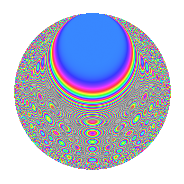# Properties

 Label 3150.2.cmLevel 3150 Weight 2 Character orbit cm Rep. character $$\chi_{3150}(107,\cdot)$$ Character field $$\Q(\zeta_{12})$$ Dimension 192 Sturm bound 1440

# Related objects

## Defining parameters

 Level: $$N$$ = $$3150 = 2 \cdot 3^{2} \cdot 5^{2} \cdot 7$$ Weight: $$k$$ = $$2$$ Character orbit: $$[\chi]$$ = 3150.cm (of order $$12$$ and degree $$4$$) Character conductor: $$\operatorname{cond}(\chi)$$ = $$105$$ Character field: $$\Q(\zeta_{12})$$ Sturm bound: $$1440$$

## Dimensions

The following table gives the dimensions of various subspaces of $$M_{2}(3150, [\chi])$$.

Total New Old
Modular forms 3072 192 2880
Cusp forms 2688 192 2496
Eisenstein series 384 0 384

## Trace form

 $$192q + 8q^{7} + O(q^{10})$$ $$192q + 8q^{7} + 96q^{16} - 48q^{22} + 8q^{28} - 32q^{31} - 16q^{37} - 32q^{43} - 8q^{58} + 112q^{61} - 32q^{67} - 64q^{73} + 32q^{76} + 32q^{82} + 24q^{88} + 144q^{91} + 208q^{97} + O(q^{100})$$

## Decomposition of $$S_{2}^{\mathrm{new}}(3150, [\chi])$$ into newform subspaces

The newforms in this space have not yet been added to the LMFDB.

## Decomposition of $$S_{2}^{\mathrm{old}}(3150, [\chi])$$ into lower level spaces

$$S_{2}^{\mathrm{old}}(3150, [\chi]) \cong$$ $$S_{2}^{\mathrm{new}}(105, [\chi])$$$$^{\oplus 8}$$$$\oplus$$$$S_{2}^{\mathrm{new}}(210, [\chi])$$$$^{\oplus 4}$$$$\oplus$$$$S_{2}^{\mathrm{new}}(315, [\chi])$$$$^{\oplus 4}$$$$\oplus$$$$S_{2}^{\mathrm{new}}(525, [\chi])$$$$^{\oplus 4}$$$$\oplus$$$$S_{2}^{\mathrm{new}}(630, [\chi])$$$$^{\oplus 2}$$$$\oplus$$$$S_{2}^{\mathrm{new}}(1050, [\chi])$$$$^{\oplus 2}$$$$\oplus$$$$S_{2}^{\mathrm{new}}(1575, [\chi])$$$$^{\oplus 2}$$

## Hecke Characteristic Polynomials

There are no characteristic polynomials of Hecke operators in the database